Learn all Concepts of Chapter 8 Class 10 (with VIDEOS). Check - Trigonometry - Class 10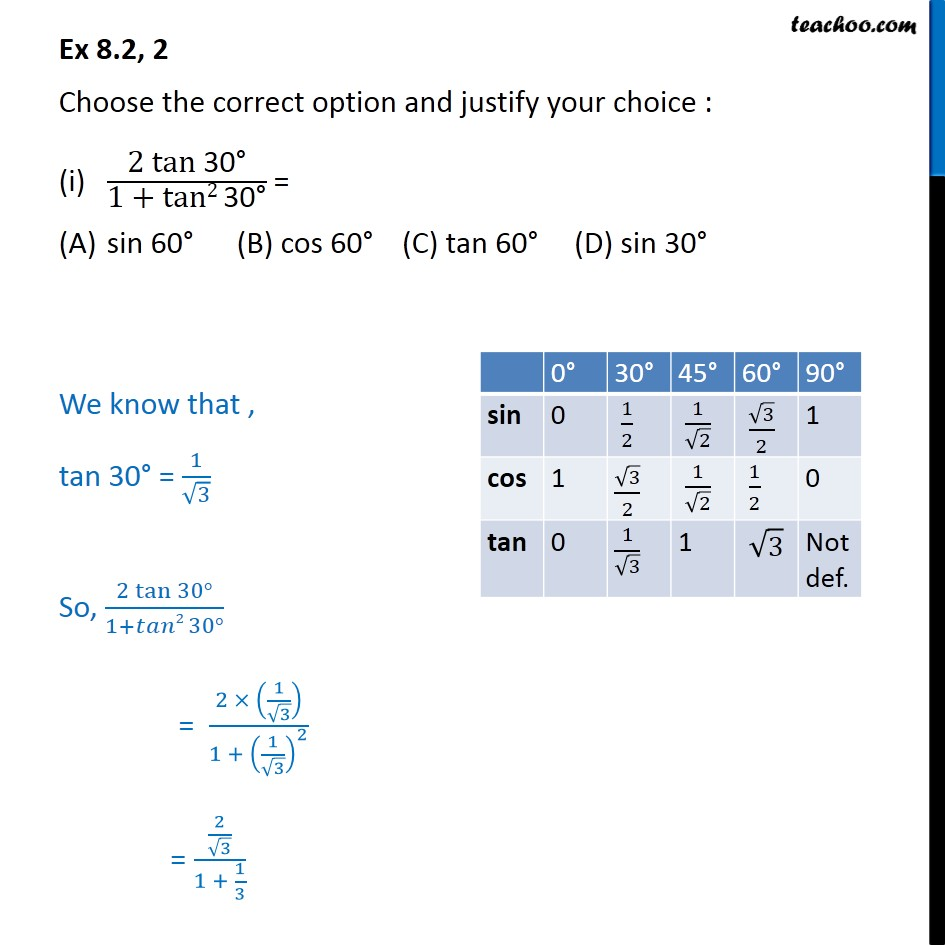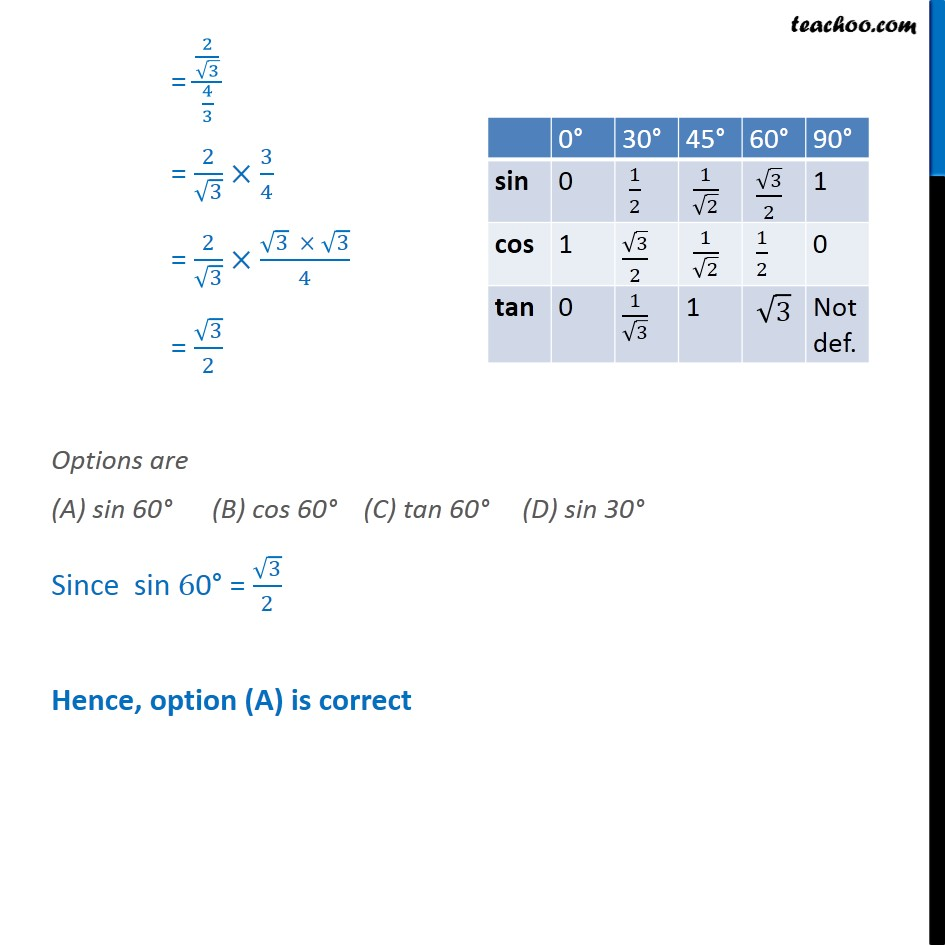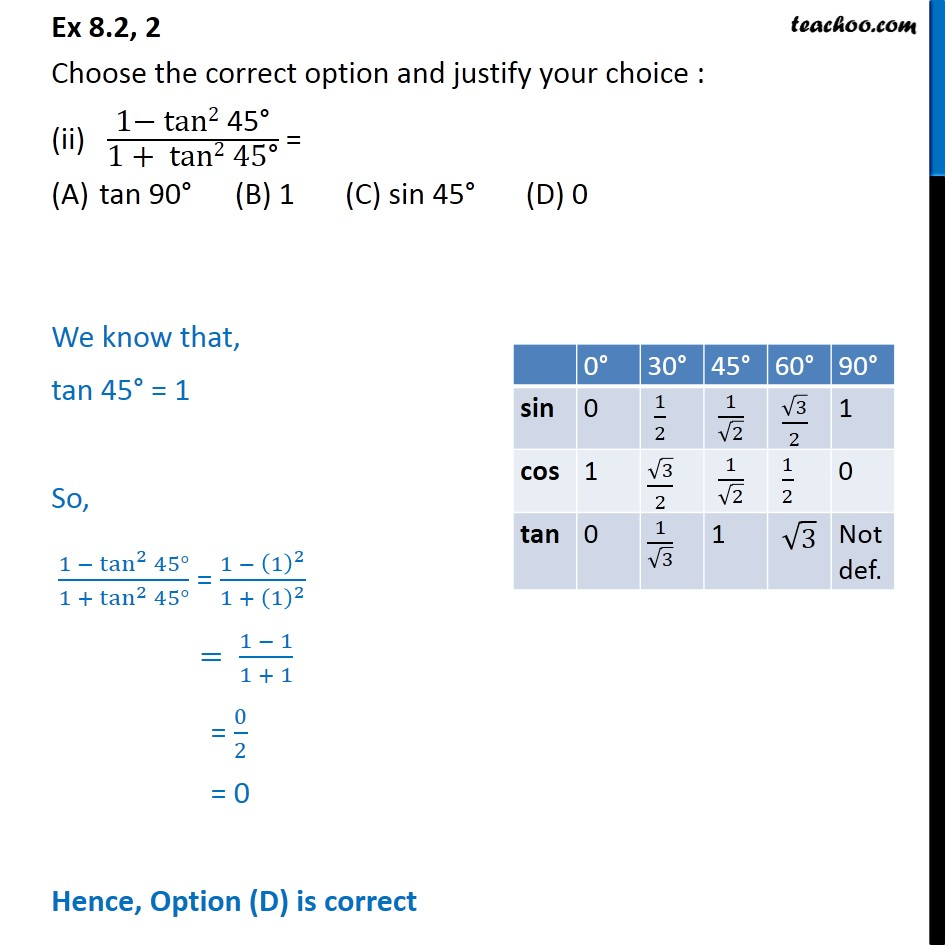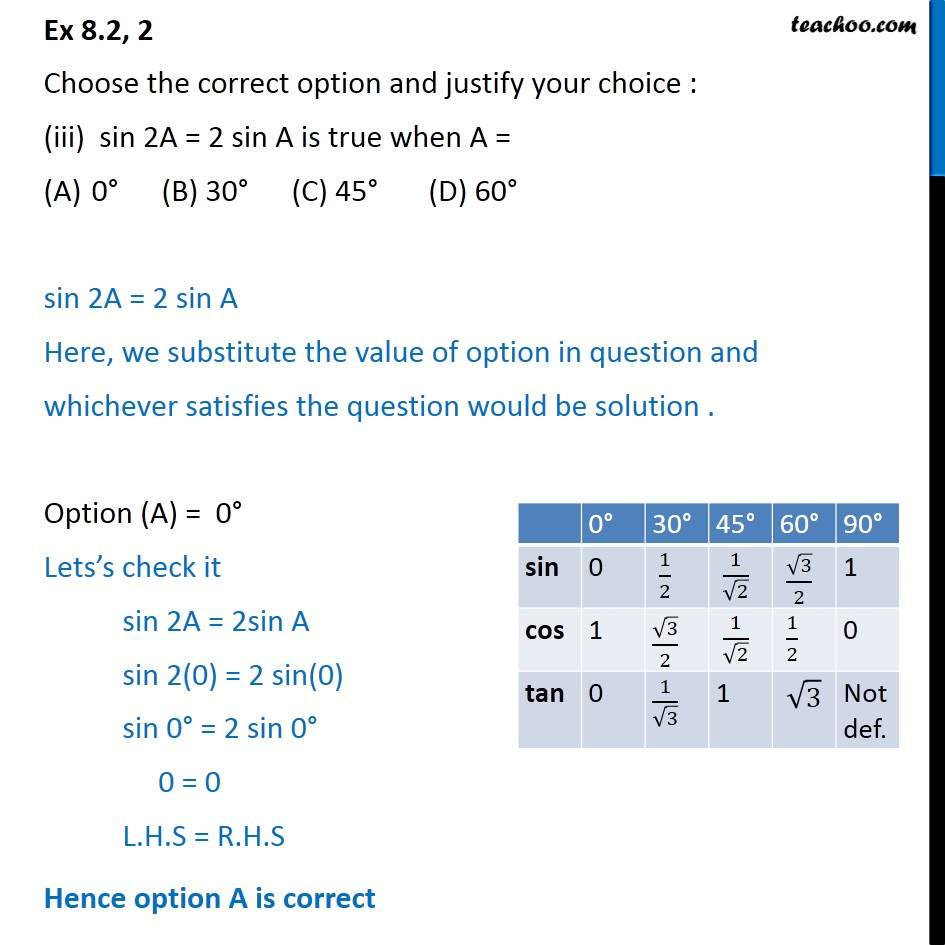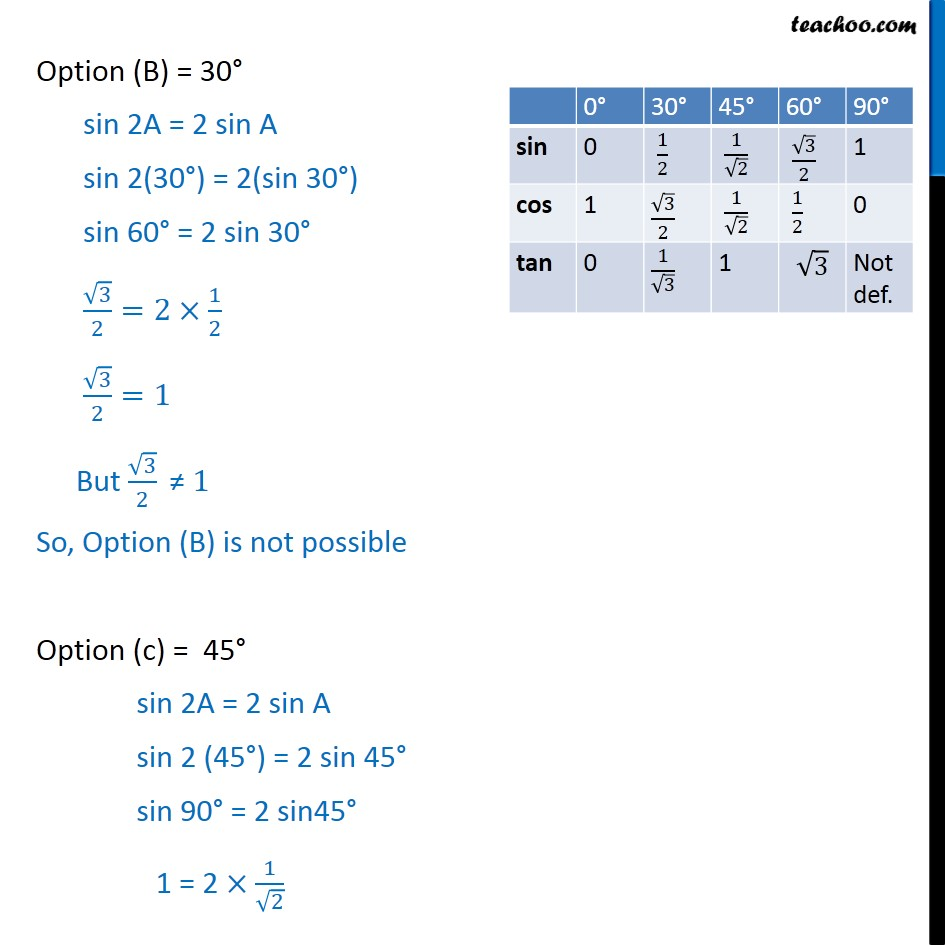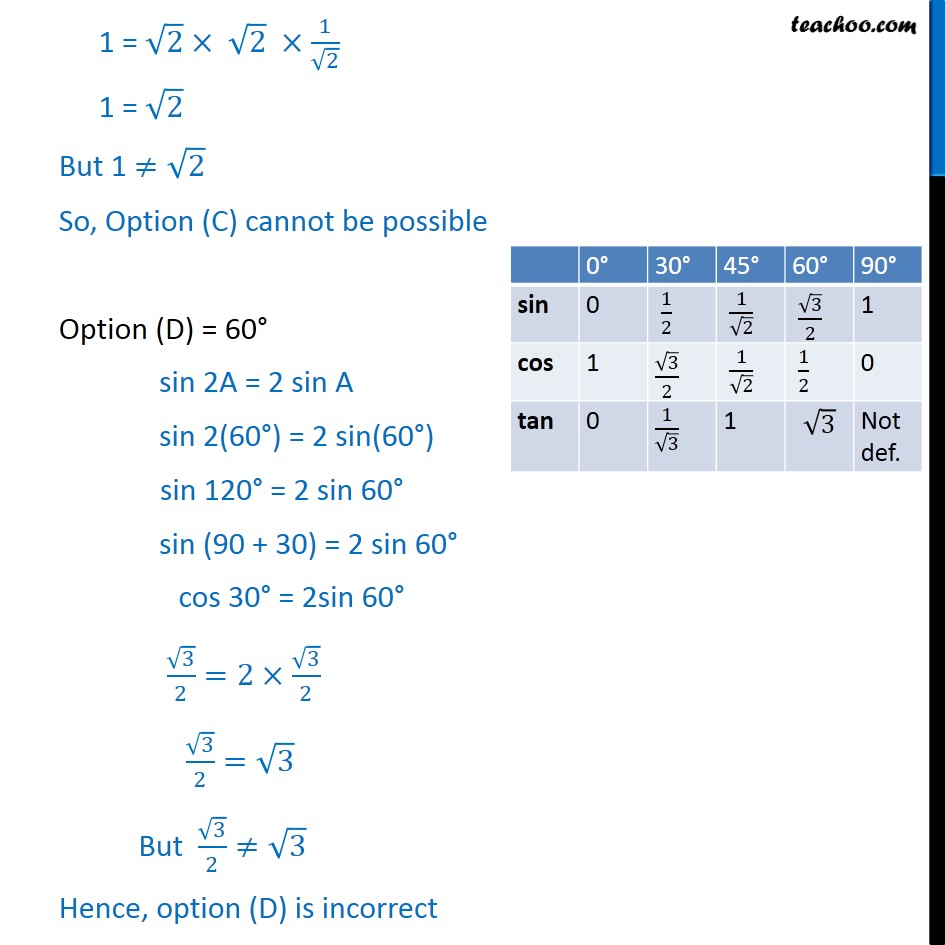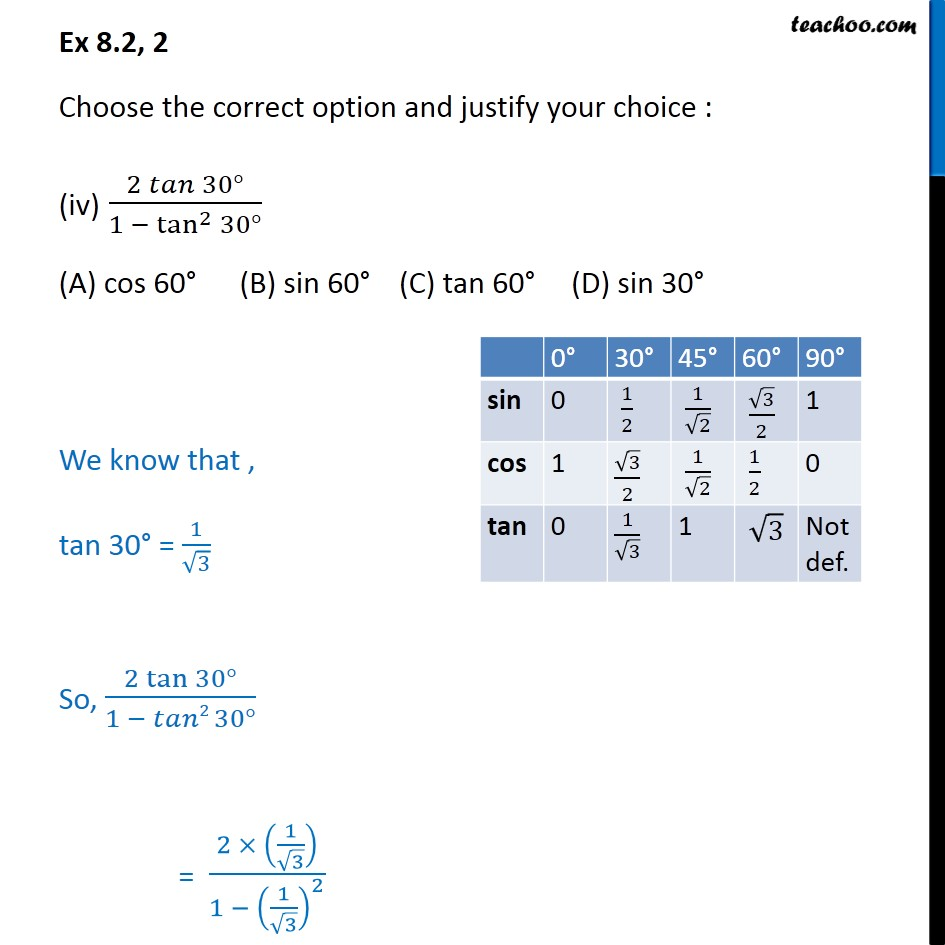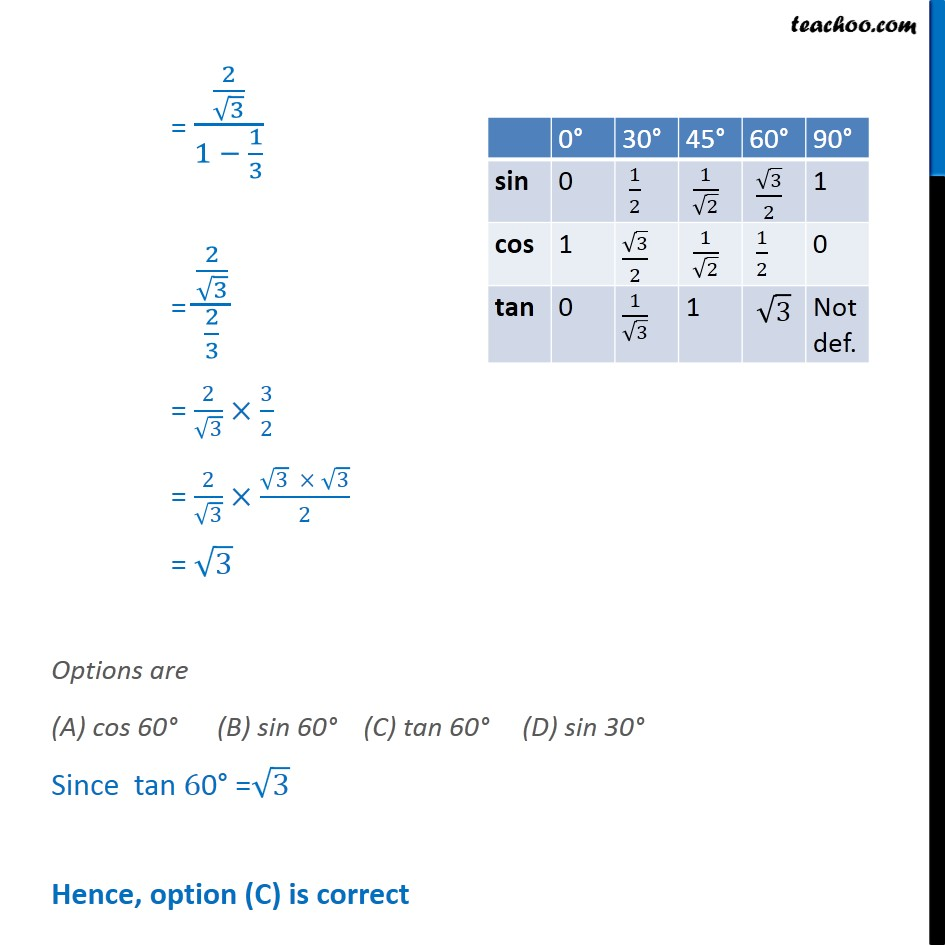1. Chapter 8 Class 10 Introduction to Trignometry
2. Serial order wise
3. Ex 8.2

Transcript

Ex 8.2, 2 Choose the correct option and justify your choice : (i) "2 tan 30 " /"1 + tan2 30 " = sin 60 (B) cos 60 (C) tan 60 (D) sin 30 We know that , tan "30 " = 1/ 3 So, (2 tan 30 )/(1+ 2 30 ) = (2 (1/ 3))/(1 + (1/ 3)^2 ) = (2/ 3)/(1 + 1/3) = (2/ 3)/(4/3) = 2/ 3 3/4 = 2/ 3 ( 3 3)/4 = 3/2 Options are (A) sin 60 (B) cos 60 (C) tan 60 (D) sin 30 Since sin "60 " = 3/2 Hence, option (A) is correct Ex 8.2, 2 Choose the correct option and justify your choice : (ii) "1 tan2 45 " /"1 + tan2 45 " = tan 90 (B) 1 (C) sin 45 (D) 0 We know that, tan 45 = 1 So, (1 tan ^2 45 )/(1 + tan ^2 45 ) = (1 (1)^2)/(1 + (1)^2 ) = (1 1)/(1 + 1) = 0/2 = 0 Hence, Option (D) is correct Ex 8.2, 2 Choose the correct option and justify your choice : (iii) sin 2A = 2 sin A is true when A = 0 (B) 30 (C) 45 (D) 60 sin 2A = 2 sin A Here, we substitute the value of option in question and whichever satisfies the question would be solution . Option (A) = 0 Lets s check it sin 2A = 2sin A sin 2(0) = 2 sin(0) sin 0 = 2 sin 0 0 = 0 L.H.S = R.H.S Hence option A is correct Option (B) = 30 sin 2A = 2 sin A sin 2(30 ) = 2(sin 30 ) sin 60 = 2 sin 30 3/2=2 1/2 3/2=1 But 3/2 " " 1 So, Option (B) is not possible Option (c) = 45 sin 2A = 2 sin A sin 2 (45 ) = 2 sin 45 sin 90 = 2 sin45 1 = 2 1/ 2 1 = 2 2 1/ 2 1 = 2 But 1 2 So, Option (C) cannot be possible Option (D) = 60 sin 2A = 2 sin A sin 2(60 ) = 2 sin(60 ) sin 120 = 2 sin 60 sin (90 + 30) = 2 sin 60 cos 30 = 2sin 60 3/2=2 3/2 3/2= 3 But 3/2 3 Hence, option (D) is incorrect So, only option (A) is correct Ex 8.2, 2 Choose the correct option and justify your choice : (iv) (2 𝑡𝑎𝑛⁡〖30°〗)/(1 − tan^2⁡〖30°〗 ) (A) cos 60° (B) sin 60° (C) tan 60° (D) sin 30° We know that , tan "30°" = 1/√3 So, (2 tan⁡〖30°〗)/(1 − 𝑡𝑎𝑛2 30°) = (2 × (1/√3))/(1 − (1/√3)^2 ) = (2/√3)/(1 − 1/3) = (2/√3)/(2/3) = 2/√3×3/2 = 2/√3×(√3 × √3)/2 = √3 Options are (A) cos 60° (B) sin 60° (C) tan 60° (D) sin 30° Since tan "60°" =√3 Hence, option (C) is correct

Ex 8.2